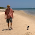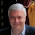## Saturday, July 21, 2018

### Questions about slave-particle mean-field theories of Hund's metals

One of most interesting new ideas about quantum matter from the last decade is that of a Hund's metal. This is a strongly correlated metal that can occurs in a multi-orbital material (model) as a result of the Hund's rule (exchange interaction) J that favours parallel spins in different orbitals.
Above some relatively low temperature (i.e. compared to the bare energy scales such as non-interacting band-widths, J, and Hubbard U) the metal becomes a bad metal, associated with incoherent excitations.
An important question concerns the extent to which slave mean-field theories can capture the stability of the Hund's metal, and its properties including the emergence of a bad metal above some coherence temperature, T*.

In a single-band Hubbard model, the strongly correlated metallic phase that occurs in proximity to a Mott insulator is associated with a small quasi-particle weight and suppression of double occupancy, reflecting suppressed charge fluctuations. This is captured by slave-boson mean-field theory, including the small coherence temperature.

In contrast, to a "Mott metal", a Hund's metal is associated with suppression of singlet spin fluctuations on different orbitals, without suppression of charge fluctuations and is seen in a Z_2 slave-spin mean-field theory at zero temperature.

Specific questions are whether slave mean-field theories at finite temperature can capture the following?
• The coherence temperature, T*.
• A suppression of spin singlet fluctuations at T increases towards T*.
• An orbital-selective bad metal may occur in proximity to an orbital selective Mott transition. This is where at least one band (orbital) is a Fermi liquid and another is a bad metal. This would mean that there are two different coherence temperatures.
• The emergence of a single low-energy scale, common in both bands, as is seen in DMFT.
• The spin-freezing temperature.
Finally, how does the stability of the Hund's metal change with the number of orbitals?
Figures in this post suggest that the Hund's physics is more pronounced with increasing the number of orbitals. However, that may be because the critical U (and thus proximity to the Mott insulator) changes with the number of orbitals and all the curves are for the same U.

1.Hi, I would like to introduce the following paper:

"Dynamical Mean-Field Theory Plus Numerical Renormalization-Group Study of Spin-Orbital Separation in a Three-Band Hund Metal"
K. M. Stadler, Z. P. Yin, J. von Delft, G. Kotliar, and A. Weichselbaum
https://doi.org/10.1103/PhysRevLett.115.136401

I wish this paper would answer some of questions on so-called "spin freezing".

1.Thanks for recommending that nice paper. I am actually reading it and hope to write something about it soon.

2.I have now written a post about the paper.

2.Ross, do you know of good review articles that introduce the paradigm of Hund's metals/physics for those that are unfamiliar? There are numerous papers for the case of Mott-type insulators, but I am unfamiliar with the Hund's coupling case.

1.Ross probably has better references, but I found the following two articles useful:

 A. Georges, L. de' Medici, and J. Mravlje, Ann Rev Condens Matter Phys 4, 137 (2013).
 L. Fanfarillo and E. Bascones, Phys Rev B 92, 075136 (2015).

2.Thanks Ray.
I think  is a very nice article and an excellent introduction.